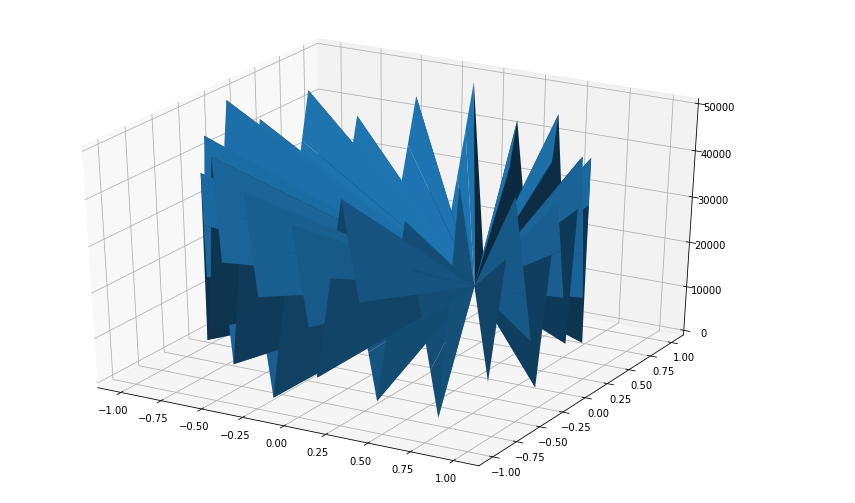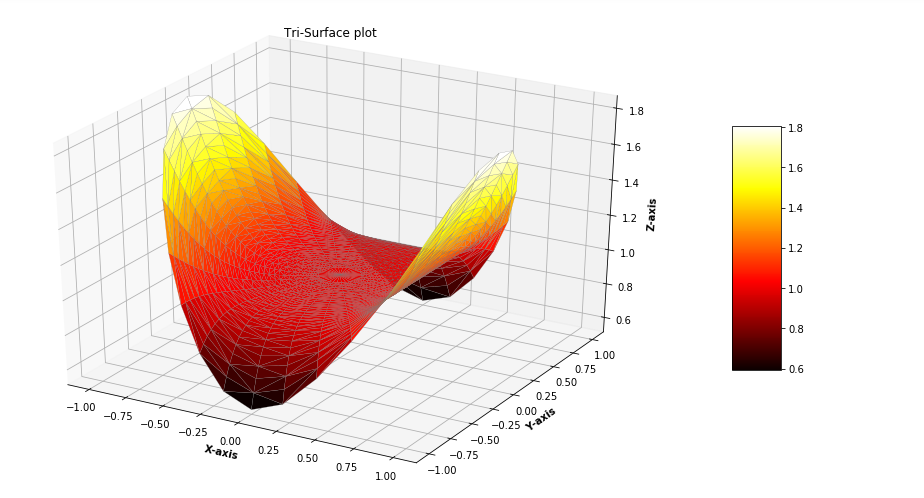# Tri-Surface Plot in Python using Matplotlib

A Tri-Surface Plot is a type of surface plot, created by triangulation of compact surfaces of finite number of triangles which cover the whole surface in a manner that each and every point on the surface is in triangle. The intersection of any two triangles results in void or a common edge or vertex. This type of plot is created where the evenly sampled grids are restrictive and inconvenient to plot. Generally Tri-Surface plots are created by calling `ax.plot_trisurf()` function of matplotlib library. Some of the attributes of the function are listed below:

Attribute Parameter
X, Y, Z dataset as 1D array to be plotted
colors color of the surface patches
cmap color map to set the color of surface patches
norm parameter to normalize map values of colors
vmin minimum value of map
vamx maximum value of map

Example 1 : Let’s create a basic Tri-Surface plot using the `ax.plot_trisurf()` function.

 `# Import libraries ` `from` `mpl_toolkits ``import` `mplot3d ` `import` `numpy as np ` `import` `matplotlib.pyplot as plt ` ` `  ` `  `# Creating dataset ` `z ``=` `np.linspace(``0``, ``50000``, ``100``) ` `x ``=` `np.sin(z) ` `y ``=` `np.cos(z)  ` ` `  `# Creating figyre ` `fig ``=` `plt.figure(figsize ``=``(``14``, ``9``)) ` `ax ``=` `plt.axes(projection ``=``'3d'``) ` ` `  `# Creating plot ` `ax.plot_trisurf(x, y, z, ` `                ``linewidth ``=` `0.2``, ` `                ``antialiased ``=` `True``); ` ` `  `# show plot ` `plt.show() `

Output :Example 2 : For better understanding Let’s take another example.

 `# Import libraries ` `from` `mpl_toolkits.mplot3d ``import` `Axes3D   ` `import` `matplotlib.pyplot as plt   ` `import` `numpy as np   ` ` `  ` `  `# Creating radii and angles ` `r ``=` `np.linspace(``0.125``, ``1.0``, ``100``)   ` `a ``=` `np.linspace(``0``, ``2` `*` `np.pi,  ` `                ``100``, ` `                ``endpoint ``=` `False``)   ` `   `  `# Repeating all angles for every radius   ` `a ``=` `np.repeat(a[..., np.newaxis], ``100``, axis ``=` `1``)   ` `   `  `# Creating datset ` `x ``=` `np.append(``0``, (r ``*` `np.cos(a)))   ` `y ``=` `np.append(``0``, (r ``*` `np.sin(a)))     ` `z ``=` `(np.sin(x ``*``*` `4``) ``+` `np.cos(y ``*``*` `4``))  ` `   `  `# Creating figure ` `fig ``=` `plt.figure(figsize ``=``(``16``, ``9``))   ` `ax ``=` `plt.axes(projection ``=``'3d'``)   ` ` `  `# Creating color map ` `my_cmap ``=` `plt.get_cmap(``'hot'``) ` `   `  `# Creating plot ` `trisurf ``=` `ax.plot_trisurf(x, y, z, ` `                         ``cmap ``=` `my_cmap, ` `                         ``linewidth ``=` `0.2``,  ` `                         ``antialiased ``=` `True``, ` `                         ``edgecolor ``=` `'grey'``)   ` `fig.colorbar(trisurf, ax ``=` `ax, shrink ``=` `0.5``, aspect ``=` `5``) ` `ax.set_title(``'Tri-Surface plot'``) ` ` `  `# Adding labels ` `ax.set_xlabel(``'X-axis'``, fontweight ``=``'bold'``)  ` `ax.set_ylabel(``'Y-axis'``, fontweight ``=``'bold'``)  ` `ax.set_zlabel(``'Z-axis'``, fontweight ``=``'bold'``) ` `     `  `# show plot ` `plt.show() `

Output :My Personal Notes arrow_drop_upCheck out this Author's contributed articles.

If you like GeeksforGeeks and would like to contribute, you can also write an article using contribute.geeksforgeeks.org or mail your article to contribute@geeksforgeeks.org. See your article appearing on the GeeksforGeeks main page and help other Geeks.

Please Improve this article if you find anything incorrect by clicking on the "Improve Article" button below.

Article Tags :

Be the First to upvote.

Please write to us at contribute@geeksforgeeks.org to report any issue with the above content.